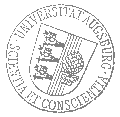# Universität AugsburgInstitut für MathematikOberseminar Differentialgeometrie

Dr. Diego Corro
KIT

spricht am

Montag, 23. Mai 2022

um

16 Uhr s.t.

im

2004/L und Zoom (hybride Veranstaltung)

über das Thema:

## »Yamabe type problems and singular Riemannian foliations«

 Abstract: The Yamabe problem is a classical problem on how to find Riemannian metrics of constant scalar curvature in a given conformal class. This problem can be written as a PDE, and positive solutions can always be found. On the other hand, the problem of finding general solutions to the Yamabe PDE turns out to be a very complicated one. A possible approach to solve in general the Yamabe PDE is to assume the presence of "symmetries" to have extra constraints in the equation. Given that group actions by isometries and geometric projections are particular types of singular Riemannian foliations, in this talk we consider singular Riemannian foliations as a form of symmetry. Using this notion of symmetry together with techniques of calculus of variations, we show how to find an infinite number of general solutions to the Yamabe problem, which are constant along the leaves of the foliation.

 Hierzu ergeht herzliche Einladung. Prof. Dr. Bernhard Hanke

Kaffee, Tee und Gebäck eine halbe Stunde vor Vortragsbeginn im Raum 2006 (L1).

[Impressum]      [Datenschutz]      wwwadm@math.uni-augsburg.de,     Mi 18-Mai-2022 16:15:37 MESZ